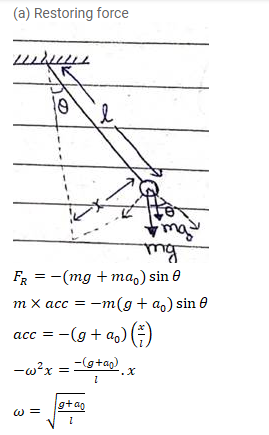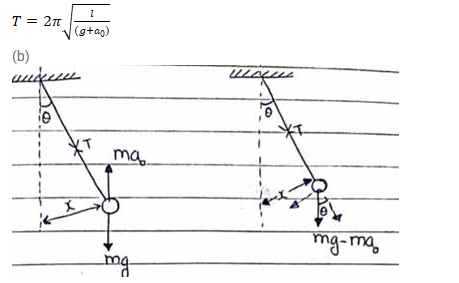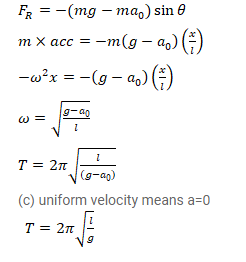# A simple pendulum of length l is suspended through the ceiling of an elevator.

Question:

A simple pendulum of length $\mathrm{I}$ is suspended through the ceiling of an elevator. Find the time period of small oscillations if the elevator

(a) is going up with an acceleration $a_{0}(\mathrm{~b})$ is going down with an acceleration $a_{0}$ and (c) is moving with a uniform velocity.

Solution: